None:
Polyps:
Strongs:For Any Prime Power

The concept of algebraic closure is of a "maximal degree extension of a field F". Every root of every irreducible polynomial is joined to F, so that a tower of fields with F at the bottom and AC at the top is created. AC is considered infinite.

Then I may assume this "maximal extension" which by choice contains every possible finite extension of F by all algebraic elements (roots of every irreducible) exists and use it to prove the following:

Theorem 1) The polynomial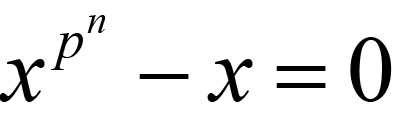(1.1)

has at most pn distinct zeroes in AC.

Proof:

First note that 0 is a root and is of multiplicity one. Then: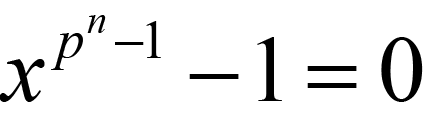(1.2)

Then also note that if a is a root then (x-a) divides the above. Then I gain g(x) by division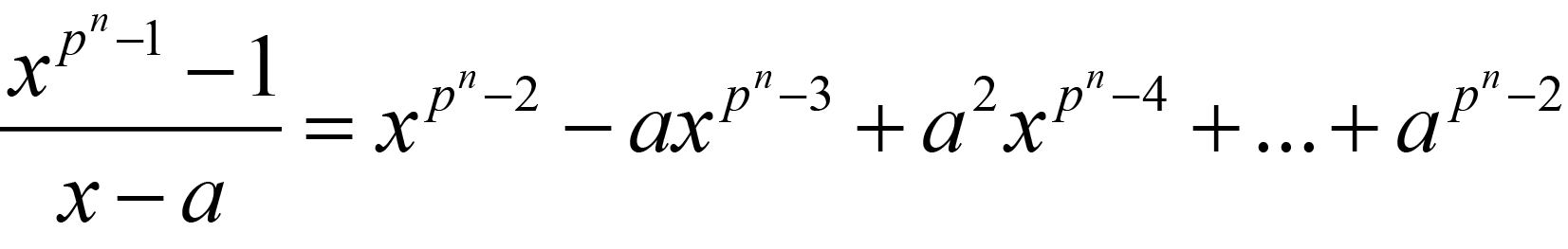(1.3)

Now, evaluating g(x) at x=a reduces every term in the polynomial above to: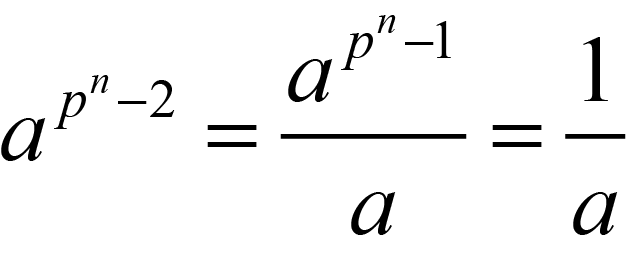(1.4)

And summing the terms modulo p I get g(a) equal to :(1.5)

Which is not zero. So, every root of (1.1) is of multiplicity one, and every root of (1.1) is distinct in AC.

Theorem 2)

There is a finite field for every prime power, that power being the order of the field.

Let AC be the algebraic closure of GF(p) and the set K be the set of zeroes of (1.1) in AC.

Let a, b be elements in K. Then, ab is in K as is a+b (c.f. the Frobenius automorphism) I.e. (a+b)y = ay + by with y=pn. Also (ab)y=ayby, so both sums and products between the elements of K are in K.

(-a)y = (-1)ay = (-1)a, and if p is the even prime then -1=1 so -a is in K (there are inverses additively). similarly, (1/a)y = 1/a as ay = a. (There are multiplicative inverses).

So K is closed under sums, differences, products, division etc. Then K is a subfield of AC and contains the pn elements we desired.

Theorem 3)

If F is a finite field, then for every degree r, there is an irreducible polynomial of degree r in F[x].

If F has pk elements then by the above, there is a subfield K over F in AC containing GF(p) and consisting of the zeroes of some equation of the form (1.1) above.

We need to show that F < K makes sense for all r. Now, every element of both F and K is a zero of some equation (1.1), so note that pkr= pk pk(r-1). Applying this repeatedly as we would the frobenius map noting that for a in F we have ay-a=0 with y= pk we see for all a in F,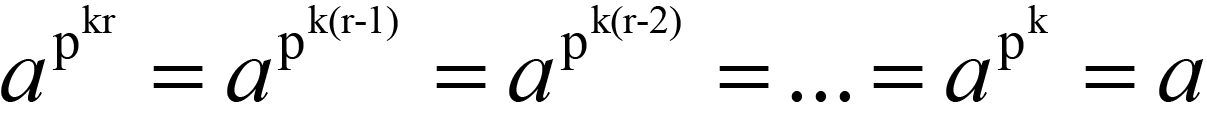(1.6)

So, F < K. Then as every polynomial in K may be represented as polynomials of degree less than r (with coefficients in F), we have the degree of K over F equal to r, by virtue of the fact that K is always a simple extension (and every root of equation (1.1) has multiplicity 1), so K = F(y) = F[x] / irr(x) where y is some root of irr(x) in F[x] with irr(x) of degree r.

Then, for any prime power there is a unique finite field of that order; and for every field of degree kr over GF(p) there is an isomorphic extension of degree r over the extension of degree k over GF(p). There is then an extension of order pkr containing that subfield of order pk in AC. Similarly, for every degree n|m there is an extension of degree m over GF(p) that contains a subfield of degree n over GF(p). Similarly if n|m then the field of order pm contains a field of order pn as a subfield.

Continue To Next Page